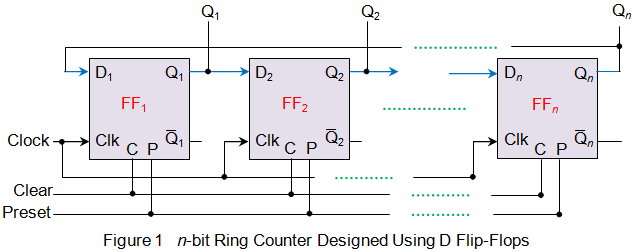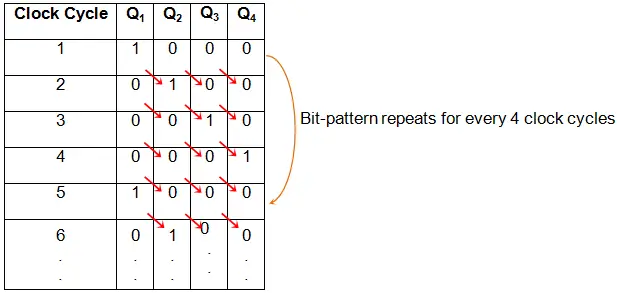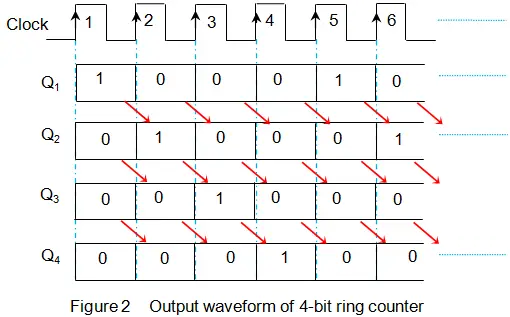# Ring Counter

A counter is nothing but a digital device meant to count. These are usually built using bi-stable devices called flip-flops. Generally either D or JK type flip-flops are used to design the counters, no matter which type they are of applies even for ring counter. In fact, the way of connection which exists between the flip-flops is the factor which determines the kind of counter designed.
Now, let us assume that we have arranged all the flip-flops in series such that the output of the preceding flip-flop is fed as an input to the immediate next flip-flop. Further, let us connect the output of the last flip-flop as an input to the first flip-flop in the chain.

The resulting circuit would be a ring counter of length equal to that of the number of flip-flops cascaded. Figure 1 shows such an arrangement made using n D flip-flops, which would thus be nothing but n-bit ring counter.Here the preset (P) pin is meant to initialize the counter by loading a certain bit-pattern into it, while the clear (C) pin is meant to clear all the flip-flops in the chain. Usually, ring counters are loaded with a pattern wherein all are zeros while a single bit will be one, for which, the ring counters are also called one-hot counters. For example, a 4-bit ring counter will be loaded with a bit pattern of 0001.
As we have the output of the last flip-flop connected back to the first flip-flop, this bit pattern continuously rotates within the counter by shifting once its position for each clock pulse fed. This kind of data movement results in repetition of bit pattern for every n clock cycles, where n is the length of the counter designed.

One example of such data movement is shown by Table I for a 4-bit ring counter, pre-fed by the bit pattern 1000. Here one can observe that the initially fed bit-pattern repeats for every 4 clock pulses. The corresponding waveforms are as shown by Figure 2.

Table I Truth Table of 4-bit Ring CounterMerit
Ring counters have only one bit high at any instant of time. This makes them readily decodable in nature unlike other counters which make use of additional logic circuitry.

Demerit
A ring counter of n-bits has only n valid states instead of 2n. This makes them inefficient in terms of state-usage.

Want To Learn Faster? 🎓
Get electrical articles delivered to your inbox every week.
No credit card required—it’s 100% free.